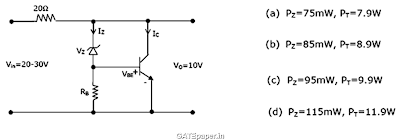GATE 2001 ECE Video Solutions on EDC (Electronic Devices and Circuits)

1. MOSFET can be used as a
a. Current controlled capacitor
b. Voltage controlled capacitor
c. Current controlled inductor
d. Voltage controlled inductor

2. The effective channel length of a MOSFET in saturation decreases with increase in
a. Gate voltage
b. Drain voltage
c. Source voltage
d. Body voltage

3. The transistor shunt regulator shown in figure has a regulated output voltage of 10 volts, when input varies from 20 volts to 30 volts. The relevant parameters for the zener diode and the transistor are: VZ = 9.5 volts, VBE = 0.3 votls, β = 99. Neglect the current throught RB. Then the maximum power dissipated in the zener diode (PZ) and the transistor (PT) are4. For the circuit shown in figure, D1 and D2 are identical diodes with ideality factor of unity. The thermal voltage VT = 25 mV.
a. If the reverse saturation current, IS, for the diode is 1 pA, then compute the current I through the circuit.
b. Calculate Vf and Vr.